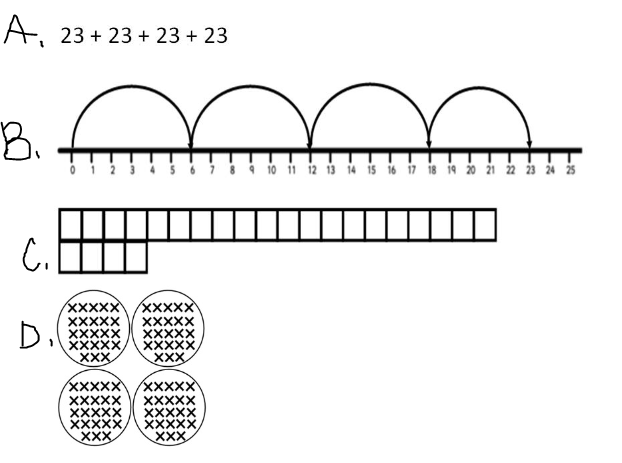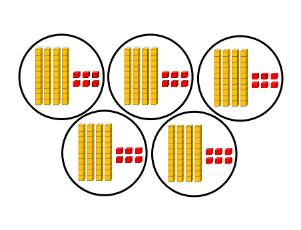Quick Facts
Fill in the Blank
Random!
Problem Solving
100

10x6

60

100

7 x ___ = 28

4

100

58x3=

174

100

Peter has 4 bags of chocolate candies. Each bag has 36 chocolate candies. How many chocolate candies does Peter have?

144

200

5x9=r

r=45

200

__ x 2 = 18

9

200

Which expression can be placed in the blank to make a true number sentence?

2 × 8 ≠ ______

A. 4 × 4

B. 16 ÷ 2

C. 20 - 4

D. 8 + 8

B. 16 ÷ 2

200

Ellis has 45 baseball cards. Danny has 5 times as many baseball cards as Ellis. How many baseball cards does Danny have?

Danny has 225 Cards.

300

70 / 10 = x

x = 7

300
25 / ___ = 5

5

300

Which two choices show 4×23?A and D

300

Lin has 4 pieces of model train track that are each 47 centimeters long. If she lines them up end to end, how long will the entire track be?

188 centimeters long

400

Select each multiplication fact that has a product of 30.

A. 5x4=

B. 3x5=

C. 3x10=

D. 5x6=

C and D

400

6 x ___ = 30

5

400

Which is best represented by this model?

A. 64 x 5

B. 45 x 6

C. 46 +5

D. 46 x 5D. 46 x 5

400

Cody ran 10 miles home. Cody ran twice as many miles as Chris. How many miles did Chris run?

Chris ran 5 miles

2 x ___ = 10

500

Identify all of the correct multiplication facts.

A. 2×6=12

B. 7⋅5=40

C. 1×6=1

D. 5⋅5=10

E. 10⋅9=90

F. 8×0=0

A. 2×6=12

E. 10⋅9=90

F. 8×0=0

500

Morgan is putting 8 pencils in each school supply kit. Morgan has 72 pencils. Which equation could be used to find the number of school supply kits Morgan can make?

A. 8 × __ = 72

B. 8 × 72 = __

C. 72 × __ = 8

D. 72 × 8 = __

A. 8 x __ = 72

500

Which number sentence is true?

A. 15 ÷ 5 ≠ 2 + 1

B. 10 - 5 ≠ 25 ÷ 5

C. 2 × 6 = 15 - 3

D. 5 × 8 = 40 + 1

C. 2x6 = 15-3

C. 12 = 12

500

There are 20 students in each 5th grade class. There are 4 5th grade classes. How many 5th grade students are there?